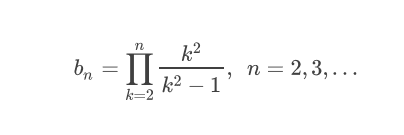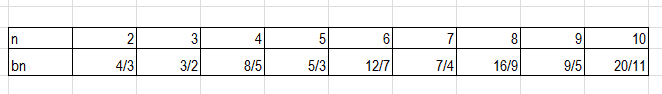# How should I simplify this product expression?

• musicgold
musicgold
Homework Statement
I need to simplify the following product expression and show it in factorial terms.
Relevant Equations
As shown below I have calculated the value of bn for 10 terms.I see that when n is an even number, the product can be represented as ## \frac {2n} {(n+1)} ##. When n is an odd number, the denominator seems to be changing and I am not able to define an expression for it.

How should I go about solving this?

Thanks

The equality also seems to hold for odd n:

##b_3 = 2.3/(3+1) = 6/4 = 3/4##
##b_5 = 2.5/(5+1) = 10/6 = 5/3##

and so on.

•musicgold
musicgold
The equality also seems to hold for odd n:

##b_3 = 2.3/(3+1) = 6/4 = 3/4##
##b_5 = 2.5/(5+1) = 10/6 = 5/3##

and so on.

Oh..I totally missed it. Thanks.
How would a trained mathematician go about analyzing this kind of question? Would they do what I did - analyzing a first few steps?

Mentor
The equality also seems to hold for odd n:

##b_3 = 2.3/(3+1) = 6/4 = 3/4##
6/4 = 3/2

•musicgold
Mentor
How would a trained mathematician go about analyzing this kind of question?
Write a few of the terms. If I start with ##P_4## (the product of the first three factors), I get
##\frac 4 3 \frac 9 8 \frac {16}{15} = \frac{2^23^34^2}{3\cdot8\cdot15}##
The numerator can already be expressed in terms of factorials, but I don't think the denominator can.

•musicgold
Staff Emeritus
Homework Helper
Gold Member
Write a few of the terms. If I start with ##P_4## (the product of the first three factors), I get
##\frac 4 3 \frac 9 8 \frac {16}{15} = \frac{2^23^34^2}{3\cdot8\cdot15}##
The numerator can already be expressed in terms of factorials, but I don't think the denominator can.
Yes, the denominator can ... well almost.

Factor the denominator. ##(k-1)(k+1)##

Write out a few terms.

•musicgold
Homework Helper
Gold Member
Oh..I totally missed it. Thanks.
How would a trained mathematician go about analyzing this kind of question? Would they do what I did - analyzing a first few steps?
There are many factors that cancel numerator and denominator. I would write down some factors and see what cancels and what is left. Probably a lot cancels and if you are lucky, all you would be left with are at the beginning and the end.

•musicgold
I would do it the way you did, and hope I saw a pattern. Of course, one then has to prove this pattern is correct. For this, you can use induction.

•musicgold
Homework Helper
Gold Member
if we take 3 successive terms we ll have
$$\frac{(k-1)(k-1)}{(k-2)k}\frac{kk}{(k-1)(k+1)}\frac{(k+1)(k+1)}{k(k+2)}$$ and after simplifications we are left with the two terms in the edges ##\frac{k-1}{k-2}## and ##\frac{k+1}{k+2}## which will get simplified by the previous and next terms so the total product will have all intermediate terms simplified to 1 and only the two outer-outer terms will survive which are the terms ##\frac{2}{1}## and ##\frac{n}{n+1}## giving the final solution $$\frac{2n}{n+1}$$

Last edited:
•musicgold and FactChecker
Staff Emeritus
Homework Helper
Gold Member
Yes, the denominator can [be written as factorials] ... well almost.

Factor the denominator. ##(k-1)(k+1)##

Write out a few terms.
Supposing you are interested in solving this with factorials, you might find it helpful to do the following prior to writing out a few terms.

Rewrite the product as follows.

##\displaystyle b_n = \prod_{k=2}^n {\ \dfrac{k^2}{k^2-1}} ##

## = \dfrac{\displaystyle \left( \prod_{k=2}^n k \right) \left( \prod_{k=2}^n k \right) }{\displaystyle \left( \prod_{k=2}^n (k-1) \right) \left( \prod_{k=2}^n (k+1) \right) } ##​

Write out a few terms, or do the general expanded expression using ellipses (...) .

•Delta2 and FactChecker
musicgold
Supposing you are interested in solving this with factorials, you might find it helpful to do the following prior to writing out a few terms.

Rewrite the product as follows.

##\displaystyle b_n = \prod_{k=2}^n {\ \dfrac{k^2}{k^2-1}} ##

## = \dfrac{\displaystyle \left( \prod_{k=2}^n k \right) \left( \prod_{k=2}^n k \right) }{\displaystyle \left( \prod_{k=2}^n (k-1) \right) \left( \prod_{k=2}^n (k+1) \right) } ##​

Write out a few terms, or do the general expanded expression using ellipses (...) .

Cool!

So if I understand this correctly, all terms except ## \left( \prod_{k=2}^n (k+1) \right) ## can be converted into factorials, because the lowest term of that product will be 3. Is that correct?

Homework Helper
Gold Member
Cool!

So if I understand this correctly, all terms except ## \left( \prod_{k=2}^n (k+1) \right) ## can be converted into factorials, because the lowest term of that product will be 3. Is that correct?
That can be converted to factorial too, it is equal to ##(n+1)!/2##

•musicgold and SammyS
musicgold
That can be converted to factorial too, it is equal to ##(n+1)!/2##
Great! Thanks